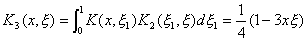[逐次逼近法]  在某种情形下，第二类Fr方程可用逐次逼近法来解。为此，设方程

`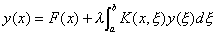(1)`

y(x)=y0(x)+y1(x)l+y2(x)l2+L                      (2)

如果级数(2)在区间[a,b]上关于x是一致收敛的，那末把它代入(1)中，可逐项积分，比较l 的系数就得到确定yn(x)的递推公式

y0(x)=F(x),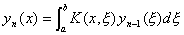(n=1,2,L)             (3)

[叠核 × 预解核 × 诺伊曼级数解]  K(x,x )为核，经递推公式

K1(x,x )=K(x,x ),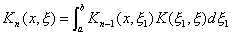(n=2,3,4,L)               (4)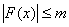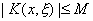时，级数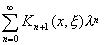k0内绝对且一致收敛，记作

`(5)``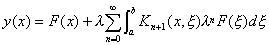(6)`

`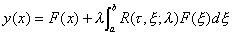，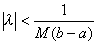(7)`

[存在性与唯一性定理]  如果把级数(5)改写为(5)上式化为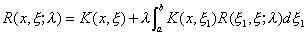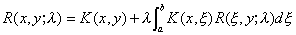举例说明预解核的实际算法。设积分方程(1)

K(x,x )=1-3xx